# Chapter 10 Hypothesis Testing What is a hypothesis

• Slides: 31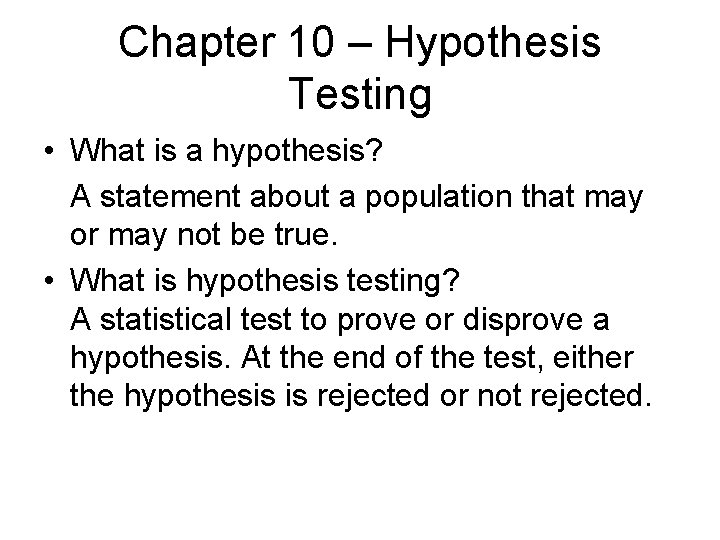Chapter 10 – Hypothesis Testing • What is a hypothesis? A statement about a population that may or may not be true. • What is hypothesis testing? A statistical test to prove or disprove a hypothesis. At the end of the test, either the hypothesis is rejected or not rejected.Steps in hypothesis testing • There are 5 steps in hypothesis testing: • Step 1 – pattern of population distribution (normal) • Step 2 – Formulation of hypothesis Null Hypothesis (H 0) Alternate Hypothesis (H 1)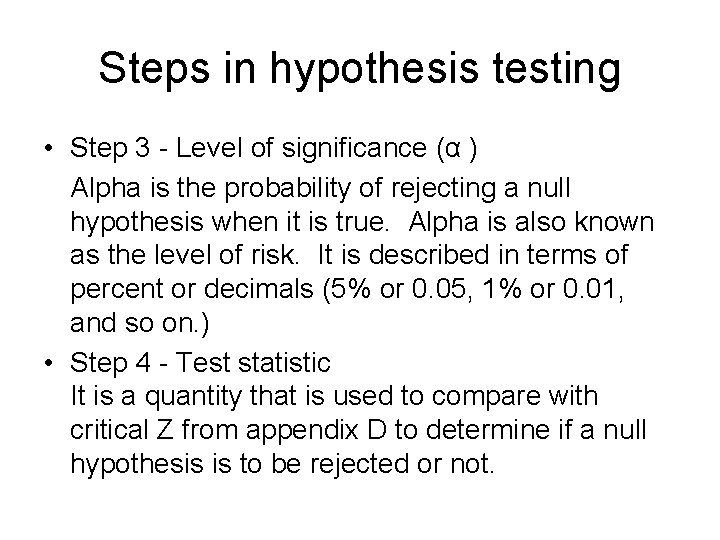Steps in hypothesis testing • Step 3 - Level of significance (α ) Alpha is the probability of rejecting a null hypothesis when it is true. Alpha is also known as the level of risk. It is described in terms of percent or decimals (5% or 0. 05, 1% or 0. 01, and so on. ) • Step 4 - Test statistic It is a quantity that is used to compare with critical Z from appendix D to determine if a null hypothesis is to be rejected or not.Steps in hypothesis testing • This quantity is the z-statistic _ Z = (x – m) / (a / Ö n). Step 5 - Decision Rule: It is a rule by which a null hypothesis is rejected or not rejected. It always takes the form: Reject H 0 if Z-statistic is > Critical Z or < - Critical Z-value is obtained from appendix D.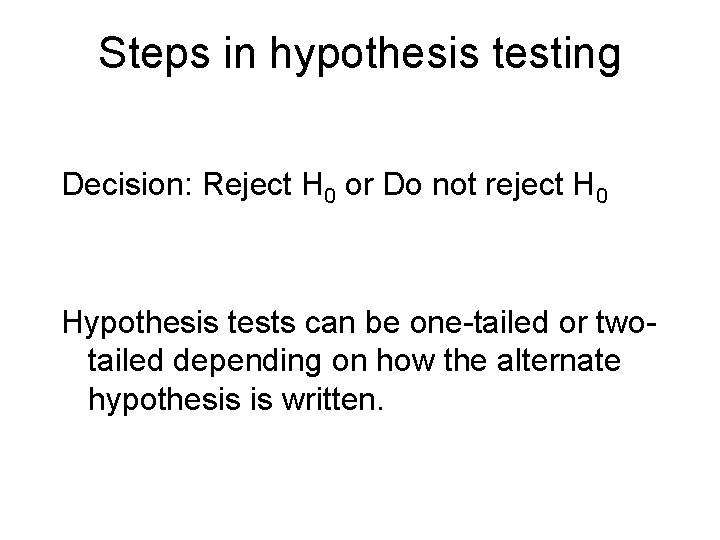Steps in hypothesis testing Decision: Reject H 0 or Do not reject H 0 Hypothesis tests can be one-tailed or twotailed depending on how the alternate hypothesis is written.Let’s take an example • Suppose: H : μ = 100 0 H : μ > 100 1 This is a one tailed test. • Another example: H : μ = 100 0 H : μ < 100 1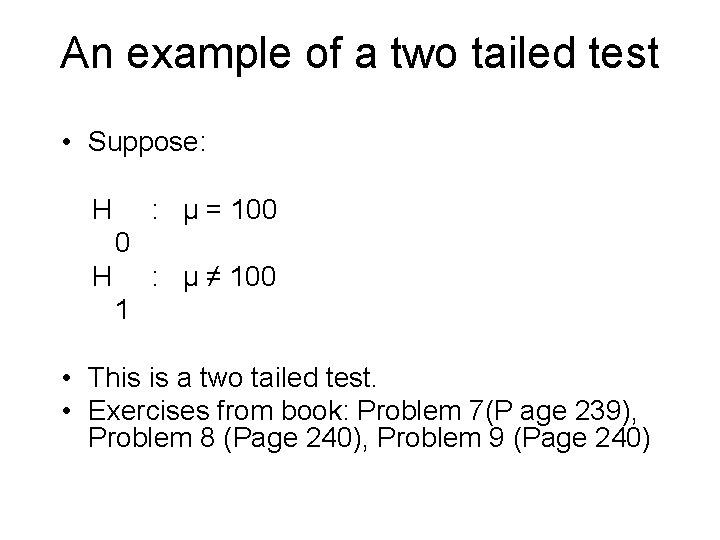An example of a two tailed test • Suppose: H : μ = 100 0 H : μ ≠ 100 1 • This is a two tailed test. • Exercises from book: Problem 7(P age 239), Problem 8 (Page 240), Problem 9 (Page 240)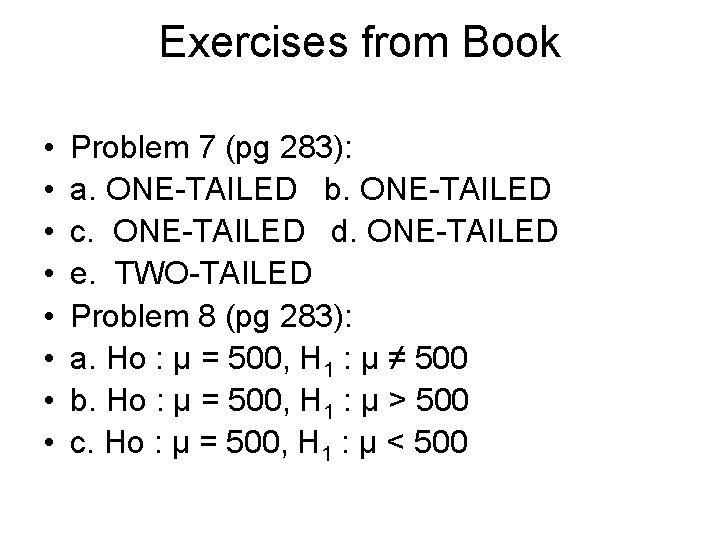Exercises from Book • • Problem 7 (pg 283): a. ONE-TAILED b. ONE-TAILED c. ONE-TAILED d. ONE-TAILED e. TWO-TAILED Problem 8 (pg 283): a. Ho : μ = 500, H 1 : μ ≠ 500 b. Ho : μ = 500, H 1 : μ > 500 c. Ho : μ = 500, H 1 : μ < 500Exercises from book • Problem 9 (pg 283) • Ho : μ = 60; H 1 : μ > 60 • Problem 10 (pg 283) • Ho : μ = 1000; H 1 : μ ≠ 1000 • Ho : μ ≤ 1000; H 1 : μ > 1000Testing for population mean • Such testing can be made under 2 situations: (1) When population standard deviation is known and (2) when population standard deviation is unknown. This chapter discusses the first. Refer to example problem 10 -2 (page 241) • Two hypotheses are: H : μ = 10 0 H : μ < 10 1Testing for population mean • Alpha ( α ) is 5% (or 0. 05). Critical Z from appendix D is – 1. 645 • Reject H 0 if Z-statistic < - 1. 645 Test statistic (Z-statistic) _ X–μ 8. 8 - 10 Z = ---------- = - 2. 0 σ/√n 2. 4 / 4Testing for population mean Decision: Reject H 0 • What does it mean? The new manufacturing process reduces tar content of cigarettes. • Example problem 10 -4.Testing for population mean H : μ = 1000 0 H : μ > 1000 1 α = 0. 025 Critical Z = 1. 96 (From Appendix D) _ X–μ 1050 - 1000 Test Statistic Z = -------------- = 2. 5 σ/n 100 / 5 Decision: Reject H 0 What does it mean? The new manufacturing process increases life of fuses.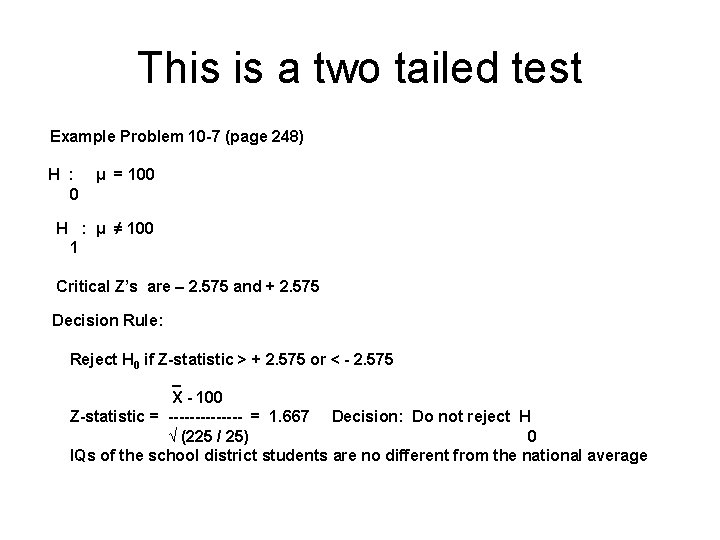This is a two tailed test Example Problem 10 -7 (page 248) H : 0 μ = 100 H : μ ≠ 100 1 Critical Z’s are – 2. 575 and + 2. 575 Decision Rule: Reject H 0 if Z-statistic > + 2. 575 or < - 2. 575 _ X - 100 Z-statistic = ------- = 1. 667 Decision: Do not reject H √ (225 / 25) 0 IQs of the school district students are no different from the national averageExample problem 13 • Problem 13 (page 251) H H 0 : μ ≥ 30 : μ < 30 1 Critical Z = - 2. 326 Decision Rule: Reject H 0 if Z-statistic ≤ - 2. 326 27 - 30 Z-Statistic = ------- = - 5. 0 6 / √ 100 Decision: Reject H 0 • What does it mean? Vendor’s claim that mean weight of his chickens is at least 30 ounces is rejected.Testing for difference between 2 means • These tests are performed when one wants to compare 2 groups of populations. • For example, is these a difference between average GPAs of students of two universities? Or, are average monthly incomes the same between two groups of people? • The testing procedure is similar to what has been presented in the previous section.Testing for difference between 2 means • The null hypothesis is always of the form: H • • : μ = μ 0 1 2 The alternative hypothesis can be either of the 3 forms: • μ > μ or 1 2 μ < μ or 1 2 μ ≠ μ 1 2Testing for difference between 2 means Formula 10 -1, p. 252 The decision rule is the same as before Example problem 10 -8 (page 252 -253) H : μ = μ H : μ ≠ μ 0 1 2 1 1 2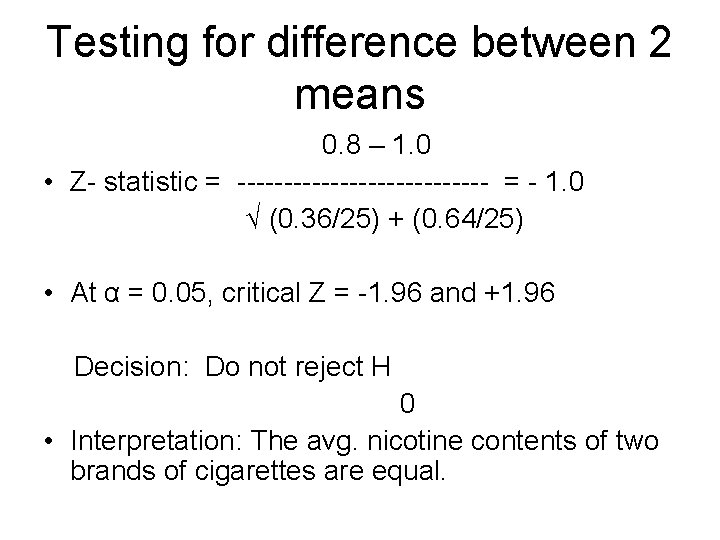Testing for difference between 2 means 0. 8 – 1. 0 • Z- statistic = -------------- = - 1. 0 √ (0. 36/25) + (0. 64/25) • At α = 0. 05, critical Z = -1. 96 and +1. 96 Decision: Do not reject H 0 • Interpretation: The avg. nicotine contents of two brands of cigarettes are equal.Problem 4, pg 255 • Is average hourly output of male workers less than that of female workers? • This is a test involving difference of means between two population groups. • Data are given in the table Ho: μ 1 = μ 2; H 1: μ 1 < μ 2 (μ 1 indicates output of males)• Z-statistic = (150 -153)/√[(70/36) + (74/36)] = -1. 5 • At α = 0. 05, critical Z is -1. 645 • Decision: Do not reject Ho • Interpretation: Average outputs of male and female workers are the same.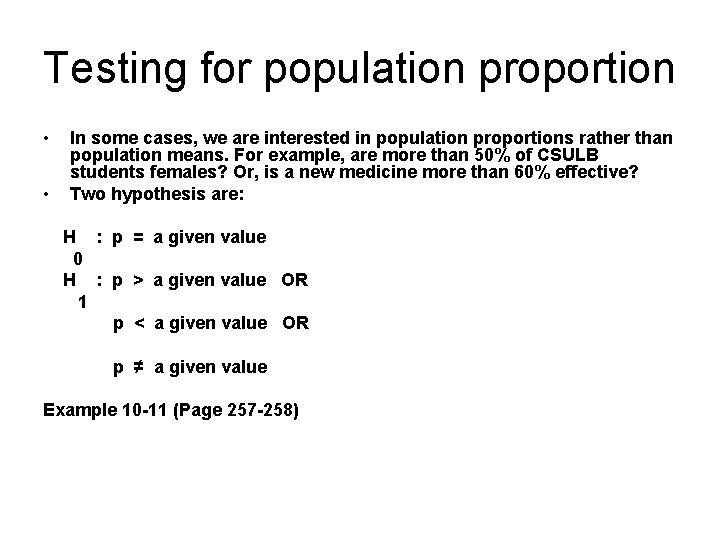Testing for population proportion • • In some cases, we are interested in population proportions rather than population means. For example, are more than 50% of CSULB students females? Or, is a new medicine more than 60% effective? Two hypothesis are: H : p = a given value 0 H : p > a given value OR 1 p < a given value OR p ≠ a given value Example 10 -11 (Page 257 -258)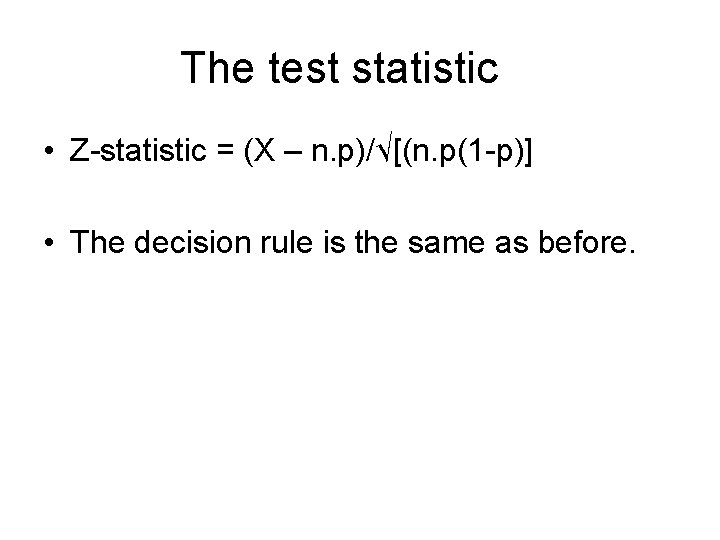The test statistic • Z-statistic = (X – n. p)/√[(n. p(1 -p)] • The decision rule is the same as before.Example problem 10 -12 (pg 259) • A TV program attracts 50% audience. A new anchorperson has been hired. Does the new hire increase audience level? Use 5% level of significance. • Ho: p = 0. 5; H 1: p > 0. 5 • Given n = 100; critical Z = 1. 645 • P = 55/100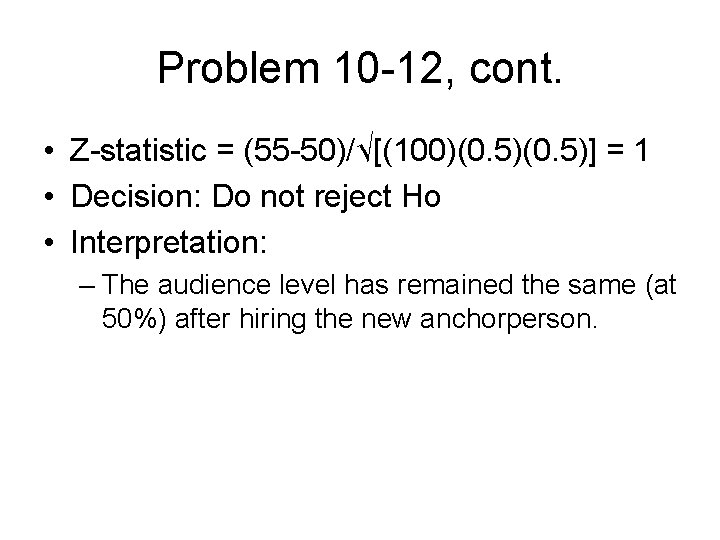Problem 10 -12, cont. • Z-statistic = (55 -50)/√[(100)(0. 5)] = 1 • Decision: Do not reject Ho • Interpretation: – The audience level has remained the same (at 50%) after hiring the new anchorperson.Problem 6, pg 261 • An auto manufacturer claims that 20% of customers prefer his products. In a sample of 100 customers, 15 indicate their preferences for his products. At 5% level of significance, can we support his claim? • Ho: p = 0. 2; H 1: p ≠ 0. 2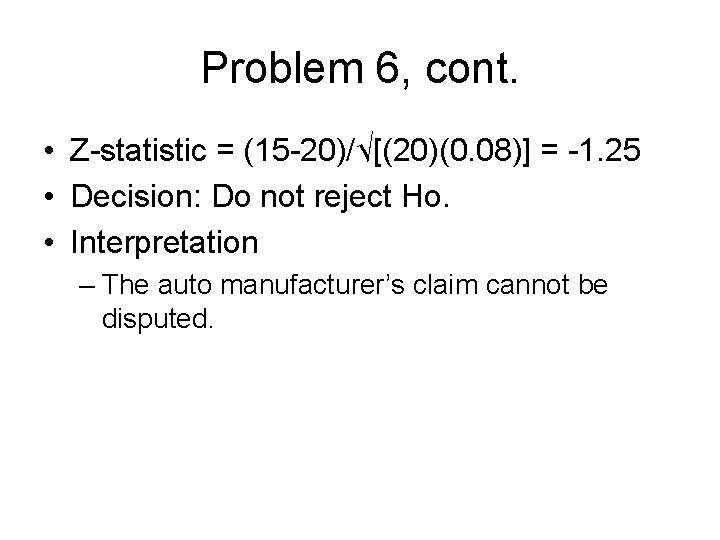Problem 6, cont. • Z-statistic = (15 -20)/√[(20)(0. 08)] = -1. 25 • Decision: Do not reject Ho. • Interpretation – The auto manufacturer’s claim cannot be disputed.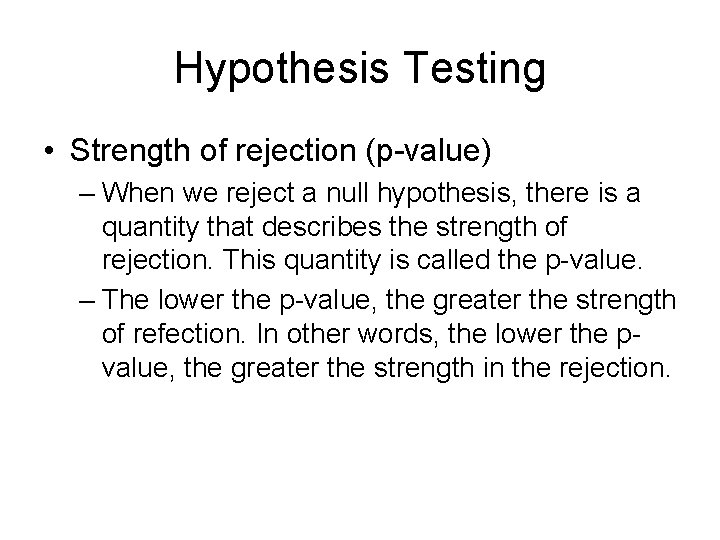Hypothesis Testing • Strength of rejection (p-value) – When we reject a null hypothesis, there is a quantity that describes the strength of rejection. This quantity is called the p-value. – The lower the p-value, the greater the strength of refection. In other words, the lower the pvalue, the greater the strength in the rejection.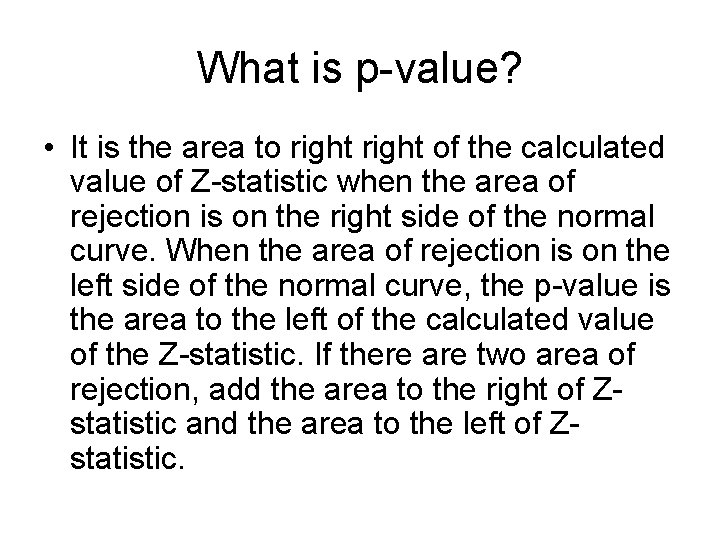What is p-value? • It is the area to right of the calculated value of Z-statistic when the area of rejection is on the right side of the normal curve. When the area of rejection is on the left side of the normal curve, the p-value is the area to the left of the calculated value of the Z-statistic. If there are two area of rejection, add the area to the right of Zstatistic and the area to the left of Zstatistic.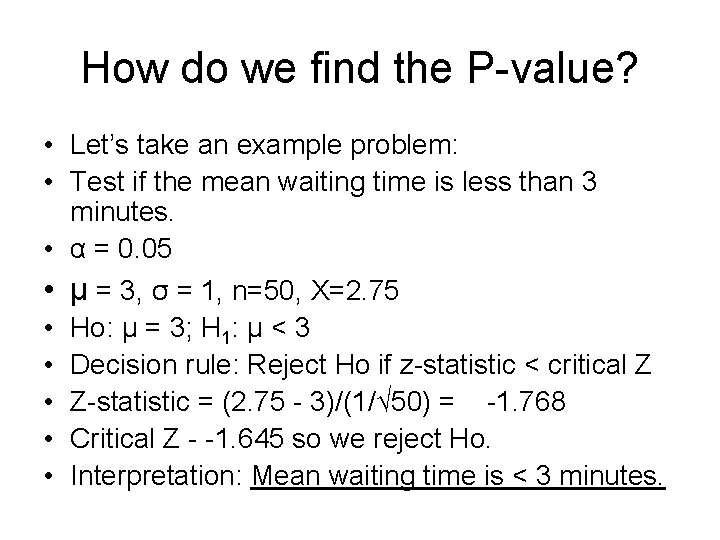How do we find the P-value? • Let’s take an example problem: • Test if the mean waiting time is less than 3 minutes. • α = 0. 05 • μ = 3, σ = 1, n=50, X=2. 75 • Ho: μ = 3; H 1: μ < 3 • Decision rule: Reject Ho if z-statistic < critical Z • Z-statistic = (2. 75 - 3)/(1/√ 50) = -1. 768 • Critical Z - -1. 645 so we reject Ho. • Interpretation: Mean waiting time is < 3 minutes.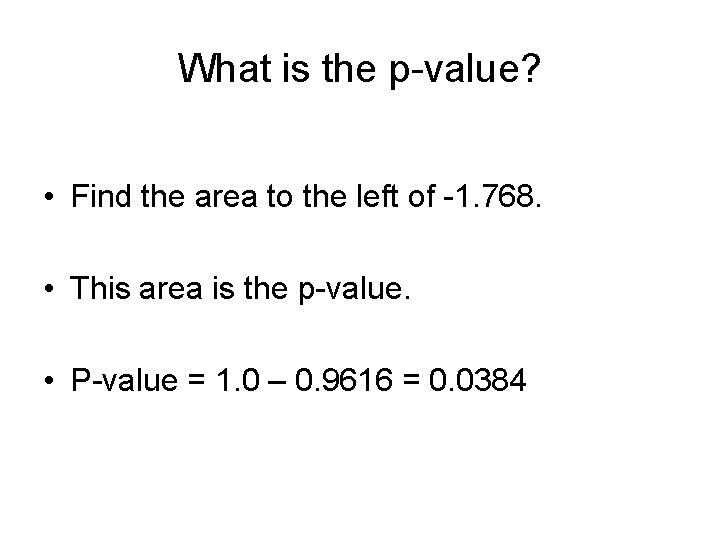What is the p-value? • Find the area to the left of -1. 768. • This area is the p-value. • P-value = 1. 0 – 0. 9616 = 0. 0384